#### Measurement of Power

Introduction to Measurement of Power:

In a DC (direct current) circuit: The power in DC circuit is provided by P = V * I,

In which V stand for = Voltage

I stand for = current.

The power can be calculated basically with the help of just Ammeter and voltmeter. The below diagram shows the circuit diagram for measuring power in DC (direct current) circuit.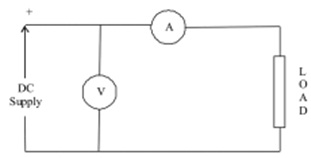Diagram - Voltmeter - Ammeter method

In 1-phase AC circuit:

Power in 1-phase AC circuit is provided by P = VICosφ,

In which,

V stands for Voltage,

I stands for current and,

Cos φ stands for power factor.

Wattmeter is employed to calculate power in AC circuits. The circuit diagram is displayed in the diagram below.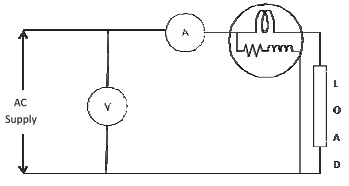Diagram - Power measurement in 1-phase AC circuit

Total power (P) = Wattmeter reading = VI Cos φ.

From this power factor can be measured as follows

Cos φ = P/ VI

3-phase AC circuit:

1) One wattmeter method: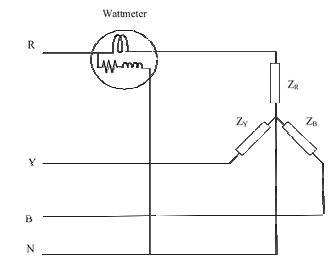Diagram - Single wattmeter method of power measurement in 3-phase circuit

For balanced 3-phase load that is ZR = ZY = ZB, single wattmeter might be connected into any one phase as displayed in the above diagram. This wattmeter will point out the power in that phase only. Because the load is balanced, the entire power in the 3-phae circuit will be calculated by

Total power = 3 *Wattmeter reading

ii) Two-wattmeter method:

This is most the general method for calculating power in a 3-phase, 3-wire system because it can be employed for both unbalanced loads (ZR ≠ ZY≠ ZB) and balanced (ZR = ZY = ZB) connected in either star/delta. The current coils are connected to any two of the lines, and the voltage coils are connected to another line, the one with no current coil connection, as displayed in the below diagram.

Total power = W1+ W2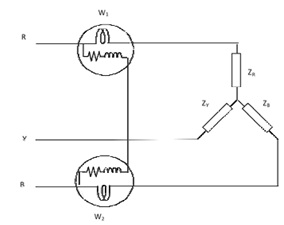Diagram - Two wattmeter method of power measurement in 3-phase circuit

iii) Three-wattmeter method:

If the load is unbalanced and the installation is 4-wire, after that 3 wattmeter are essential. The connection of wattmeter is displayed in the below diagram. Each wattmeter calculates the power in one phase and the total power will be calculated by

Total power = W1 + W2+ W3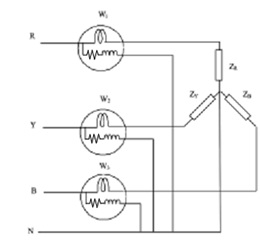Diagram: Three wattmeter method of power measurement in 3-phase circuit

Latest technology based Electrical Engineering Online Tutoring Assistance

Tutors, at the www.tutorsglobe.com, take pledge to provide full satisfaction and assurance in Measurement of Power homework help via online tutoring. Students are getting 100% satisfaction by online tutors across the globe. Here you can get homework help for Measurement of Power, project ideas and tutorials. We provide email based Measurement of Power homework help. You can join us to ask queries 24x7 with live, experienced and qualified online tutors specialized in Measurement of Power. Through Online Tutoring, you would be able to complete your homework or assignments at your home. Tutors at the TutorsGlobe are committed to provide the best quality online tutoring assistance for Electrical Engineering homework help and assignment help services. They use their experience, as they have solved thousands of the Electrical Engineering assignments, which may help you to solve your complex issues of Measurement of Power. TutorsGlobe assure for the best quality compliance to your homework. Compromise with quality is not in our dictionary. If we feel that we are not able to provide the homework help as per the deadline or given instruction by the student, we refund the money of the student without any delay.